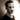# On differentiation

The muddy parts

Let's talk math. Specifically, calculating derivatives through differentiation. I've been taking a calculus course at my local university lately, and one of the things that's come up and bit me a couple times is how a lot of the reference material we're given for differentiation shows off differentiation in a way that isn't entirely clear. So in this post I want to record for the future me just how differentiation works.

## Implicit derivatives

When the common derivatives are listed, they're listed for the variable $x$, and the fact that you'll need to apply the chain rule ($(f \circ g)' = (f ' \circ g)g'$ or $(f(g(x)))' = f'(g(x)g'(x)$) when working with expressions is not really made clear. For instance, let's look at the derivative for natural logarithms: $$(\ln |x|)' = \frac{1}{x}$$

This works great for a single variable $x$, because the derivative of $x$ is $1$. However, in cases where $x$ is an expression ($2x$, $x^2$, $\sin x$, ...), you'll need to multiply that fraction with the derivative of the expression $x$. For this reason, I think it'd be much clearer if the formula sheet had it listed like this: $$(ln |x|)' = \frac{x'}{x}$$

Similarly, this also goes for other derivatives, like sines, cosines, powers of $e$, and so on:

$$(\arcsin x)' = \frac{x'}{\sqrt{1 - x^2}}$$Thomas Heartman is a developer, writer, speaker, and one of those odd people who enjoy lifting heavy things and putting them back down again. Preferably with others. Doing his best to gain and share as much knowledge as possible.